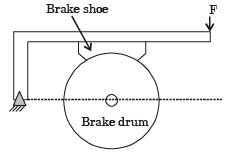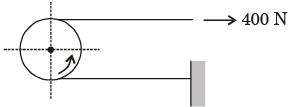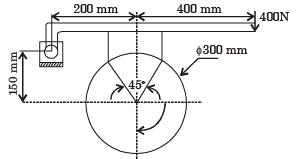Courses

# Past Year Questions: Brakes, Clutches, and Ropes Notes | EduRev

## Mechanical Engineering : Past Year Questions: Brakes, Clutches, and Ropes Notes | EduRev

The document Past Year Questions: Brakes, Clutches, and Ropes Notes | EduRev is a part of the Mechanical Engineering Course Machine Design.
All you need of Mechanical Engineering at this link: Mechanical Engineering

Question 1:A wire rope is designated as 6 x 19 standard hoisting. The numbers 6 × 19 represent



Question 2:A clutch has outer and inner diameters 100 mm and 40 mm respectively. Assuming a uniform pressure of 2 MPa and coefficient of friction of liner material 0.4, the torque carrying capacity of the clutch is



Question 3:A disk clutch is required to transmit 5 kW at 2000 rpm. The disk has a friction lining with coefficient of friction equal to 0.25. Bore radius of friction lining is equal to 25 mm. Assume uniform contact pressure of 1 MPa. The value of outside radius of the friction lining is



Question 4:Axial operation claw clutches having selflocking tooth profile



Question 5:For the brake shown in the figure, which one of the following is TRUE?[2016,Set-2]

Question 6:A force of 400 N is applied to the brake drum of 0.5 m diameter in a band brake system as shown in figure, where the wrapping angle is 180°. If the coefficient of friction between the drum and the band is 0.25, the braking torque applied, in Nm isQuestion 7:A band brake having bandwidth of 80 mm, drum diameter of 250 mm, coefficient of friction of 0.25 and angle of wrap of 270 degrees is required to exert a friction torque of 1000 N m.The maximum tension (in kN) developed in the band is



Question 8:A block-brake shown below has a face width of 300 mm and a mean coefficient of friction of 0.25. For an activating force of 400 N, the braking torque in Nm isQuestion 9:In a band brake the ratio of tight side band tension to the tension on the slack side is 3. If the angle of overlap of band on the drum is 180°, the coefficient of friction required between drum and the band is



Offer running on EduRev: Apply code STAYHOME200 to get INR 200 off on our premium plan EduRev Infinity!

## Machine Design

57 videos|71 docs|102 tests

,

,

,

,

,

,

,

,

,

,

,

,

,

,

,

,

,

,

,

,

,

,

,

,

,

,

,

;